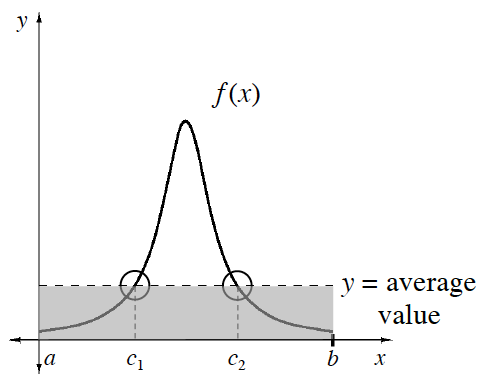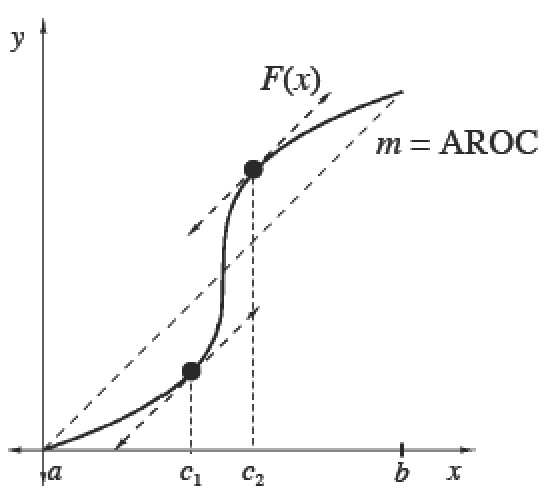### Home > APCALC > Chapter 8 > Lesson 8.3.2 > Problem8-118

8-118.

A particle is moving along the $y$-axis with a position function $y(t) =\frac { 1 } { 2 }t^2 + 2t + 1$. Determine all times when the particle is traveling at its average rate between $0$ and $8$ seconds.

You are being asked to find all times that the actual velocity is the same as the average velocity on the interval $[0, 8]$. You are given the position function. How will you find the actual velocity function? How will you find the average velocity on $[0, 8]$?

AROC = IROC somewhere

$\frac{y(8)-y(0)}{8-0}=v(c)$

Solve for $c$.

#### The Mean Value Theorem

 The Mean Value Theorem for IntegralsIf $f$  is continuous on $[a, b]$, then there exists at least one point $x = c$ in $\left(a, b\right)$ such that                     $\frac { 1 } { b - a } \int _ { a } ^ { b } f ( x ) d x= f(c)$.The Mean Value Theorem for DerivativesIf $F$ is continuous on $[a, b]$ and differentiable on $\left(a, b\right)$, then there exists at least one point $x = c$ in $\left(a, b\right)$ such that $F ^ { \prime } ( c ) = \frac { F ( b ) - F ( a ) } { b - a } = f ( c )$.